ELF/MAGIC, ELFIN, and Magnetic Moment Methods are types of Integral Equation Methods.
There are many similarities, so we will explain them in the following order. It gradually becomes more complex.

① ELFIN, for 1 element (1 variable, 1 charge) .
② ELFIN, for 2 elements (2 variables, 2 charges) .
③ Magnetic Moment Method for 1 variable, 1 element (1 variable, 2 magnetic charges) .
④ Magnetic Moment Method for 1 variable, 2 elements (2 variables, 4 magnetic charges) .
⑤ ELF/MAGIC, 6 variables per element, 6 magnetic charges .

## The ELF series solves problems by the following procedure.

[ ELFIN ]
１．The source of the electric field is the variable. Variables can be electric charge, polarization, etc.
２．Equations are formulated using Coulomb's law of electric potential and electric field and the equation relating DE curves.
３．Solve the equation to determine the source of the electric field.
４．The electric field created by the source is calculated using Coulomb's law.

[ ELF/MAGIC ]
１．The source of the magnetic field is the variable. Variables can be magnetic charges, magnetic moments, eddy currents, etc.
２．Equations are formulated using Coulomb's law of magnetic fields, Biot-Savart's law, Faraday's law and the relational equations for BH curves.
３．Solve the equation to determine the source of the magnetic field.
４．The magnetic field created by the source is calculated using Coulomb's law of magnetic fields and Biot-Savart's law.

## For electric potential (electric field) (ELFIN)

Here is an example of three electrodes.
Suppose that each potential V is specified at the center of each element.
Assume that the geometry of each element is a rectangle and that each charge density σ is uniform within each plane.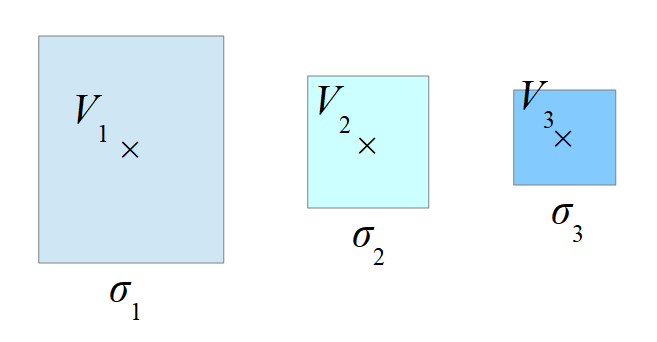### ①ELFIN, for one element

The magnitude of the potential V1 at the center position of the elements is proportional to the charge density σ1.The proportionality constant G11 can be calculated by the following integral formula
(The integration is done on the face of the element.)Dividing V1 by G11 gives the charge density σ1.### ②ELFIN, for two elements

We also add the potential due to the charge of the second element.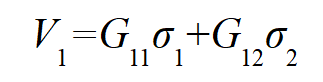The proportionality constant G12 can be calculated by the following integral formula
(The integration is done on the face of the second element.)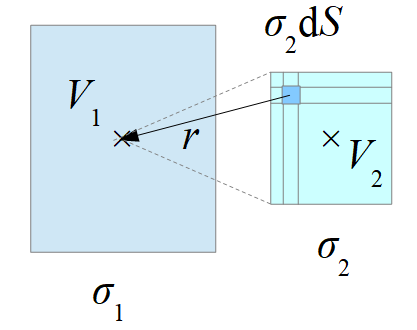#### The same equation can be formulated for V2.

This gives the following simultaneous linear equations(σ1, σ2) are unknown values and (V1, V2) are specified potentials.
Solving the equation gives the charge densities (σ1, σ2) of the elements.
Once the charge density is determined, the electric field and potential at any given location can be calculated.

The same calculation can be performed when there are many variables.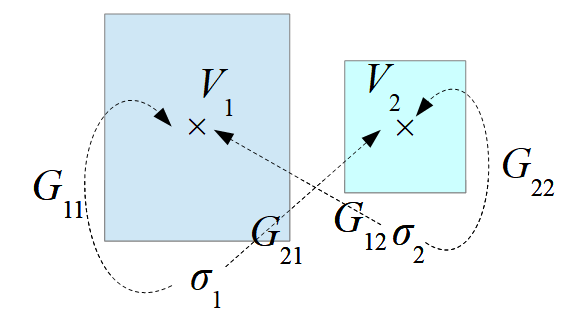## For magnetic fields (Magnetic Moment Method)

Here is an example of three magnetic elements.
Suppose there are magnetic elements in an external magnetic field H0.
The geometry of each element is rectangular, and the magnetic moment M of each element is assumed to be uniform in each element.
The magnetic moment M is the magnetic field H at the center of the elements times the magnetic susceptibility χ.

### ③Magnetic Moment Method for one variable, one element

Calculate the magnetic field created by the magnetic moment M1 of the element.
First, replace M1 with an equivalent surface magnetic charge σ1 .
σ1 = M1・n (n is the unit normal vector.)
The magnetic field created by the surface magnetic charge is calculated by Coulomb's law.

The magnetic moment M1 of an element generates an antimagnetic field h11 inside the element.The proportionality constant G11 can be calculated by the following integral formula
(The integration is done on two faces of the elements in the case of a rectangular body.)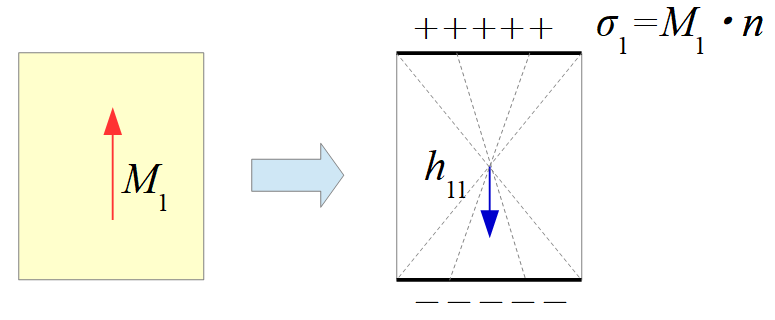#### The magnetic field H1 inside the element can be expressed as the sum of the antimagnetic field and the external magnetic field.Multiplying the magnetic field by the magnetic susceptibility gives the magnetic moment.Substitution gives the equation for M1.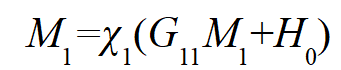Solving for M1 gives the magnetic moment.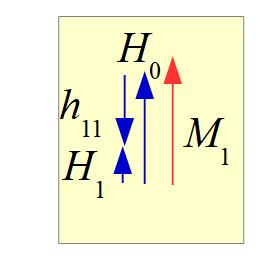### ④Magnetic Moment Method for one variable, two elements

We also add the magnetic field h12 due to the magnetic moment of the second element.The same is true for H2.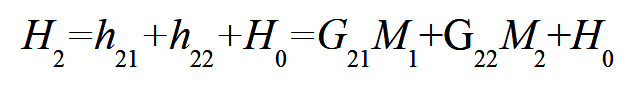#### Multiplying the magnetic field by the magnetic susceptibility gives the magnetic moment.Substitution gives simultaneous equations for (M1, M2).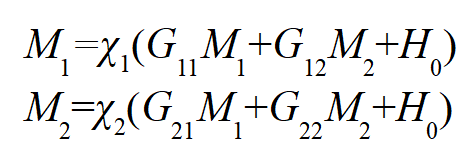Solving the simultaneous equations gives the magnetic moments (M1, M2) of the elements.
Once the magnetic moments are determined, the magnetic field at any location can be calculated.

The same calculation can be performed when there are many variables.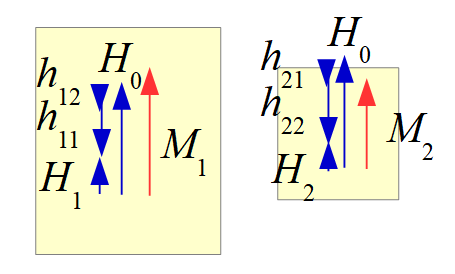### ⑤Magnetic Moment Method to ELF/MAGIC

The method of solving by assuming that the magnetic moment is uniform within an element is called the Magnetic Moment Method.
The number of variables in the elements is 3 since there are 3 components in the X, Y, and Z directions, and the magnetic charge is 6.
In this method, the magnetic moment does not bend in the elements and the magnetic flux travels straight.
In ELF/MAGIC, the number of variables per element is set to 6, the same as the number of faces.
This allows the magnetic flux to bend freely.

#### In ELF/MAGIC, the magnetic flux bends or spreads within the elements and flows freely as shown in the following figure.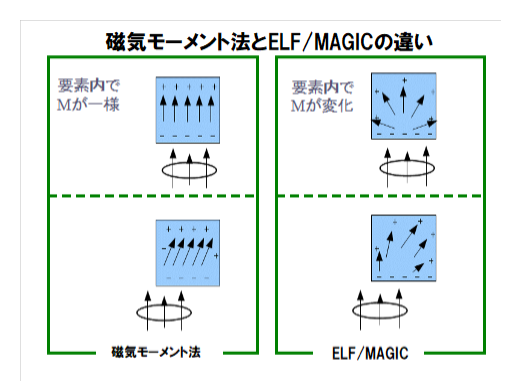## Notes on Mesh Division

The FEM requires that elements share nodes at their joint surfaces.
On the other hand, the Magnetic Moment Method and ELF/MAGIC do not require nodes to be shared.
The magnetic flux flows at the junction surface due to the near cancellation of the surface magnetic charge.
Therefore, it is also possible to divide without sharing nodes, as shown in and below.

However, since the magnetic charge density on one surface is uniform, when it is divided like , the surface magnetic charge that is not canceled appears on the surface of the magnetic body, and the magnetic flux leaks largely from there into the air.

And the magnetic flux flowing inside the magnetic body will be reduced.
division is more accurate.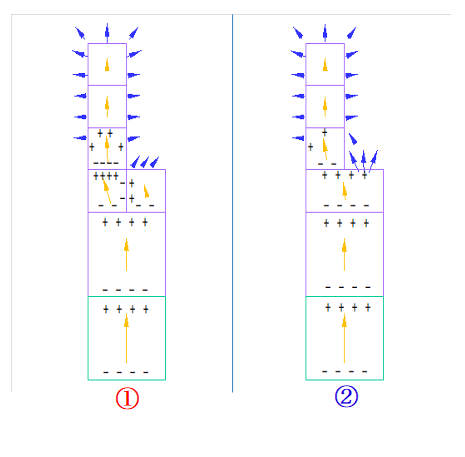a:1920 t:2 y:0